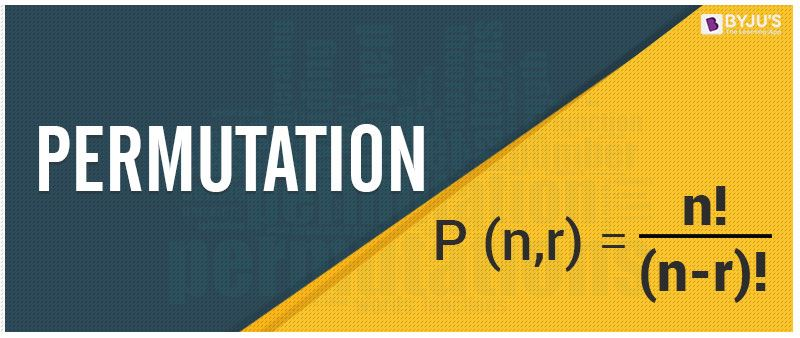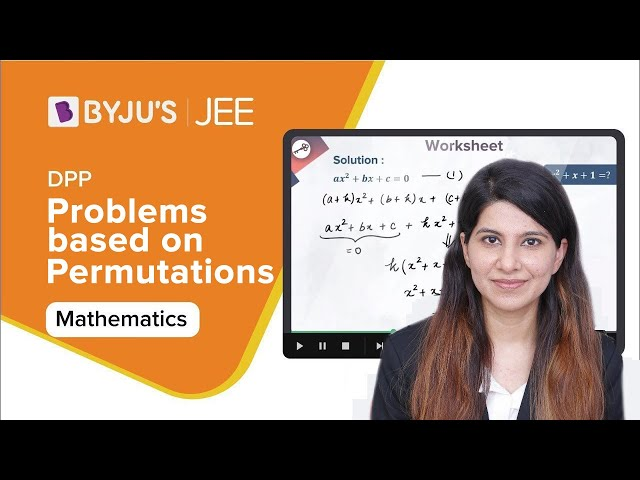# Permutation

A permutation is an arrangement of objects in a definite order. The members or elements of sets are arranged here in a sequence or linear order. For example, the permutation of set A={1,6} is 2, such as {1,6}, {6,1}. As you can see, there are no other ways to arrange the elements of set A.

In permutation, the elements should be arranged in a particular order whereas in combination the order of elements does not matter. Also, read: Permutation And Combination

When we look at the schedules of trains, buses and the flights we really wonder how they are scheduled according to the public’s convenience. Of course, the permutation is very much helpful to prepare the schedules on departure and arrival of these. Also, when we come across licence plates of vehicles which consists of few alphabets and digits. We can easily prepare these codes using permutations.## Definition of Permutation

Basically Permutation is an arrangement of objects in a particular way or order. While dealing with permutation one should concern about the selection as well as arrangement. In Short, ordering is very much essential in permutations. In other words, the permutation is considered as an ordered combination.

## Representation of Permutation

We can represent permutation in many ways, such as:

• $$\begin{array}{l}\large \mathbf{P(n,k)}\end{array}$$
• $$\begin{array}{l}\large \mathbf{P^{n}_{k}}\end{array}$$
• $$\begin{array}{l}\large \mathbf{_{n}P_{k}}\end{array}$$
• $$\begin{array}{l}\large \mathbf{^{n}P_{k}}\end{array}$$
• $$\begin{array}{l}\large \mathbf{P _{n}\, _{,k}}\end{array}$$

## Formula

The formula for permutation of n objects for r selection of objects is given by: P(n,r) = n!/(n-r)!
For example, the number of ways 3rd and 4th position can be awarded to 10 members is given by:
P(10, 2) = 10!/(10-2)! = 10!/8! = (10.9.8!)/8! = 10 x 9 = 90

## Types of Permutation

Permutation can be classified in three different categories:

• Permutation of n different objects (when repetition is not allowed)
• Repetition, where repetition is allowed
• Permutation when the objects are not distinct (Permutation of multi sets)

Let us understand all the cases of permutation in details.

### Permutation of n different objects

If n is a positive integer and r is a whole number, such that r < n, then P(n, r) represents the number of all possible arrangements or permutations of n distinct objects taken r at a time. In the case of permutation without repetition, the number of available choices will be reduced each time. It can also be represented as: nPr.

P(n, r) = n(n-1)(n-2)(n-3)……..upto r factors

P(n, r) = n(n-1)(n-2)(n-3)……..(n – r +1)

$$\begin{array}{l}\large \Rightarrow P(n,r) = \frac{n!}{(n-r)!}\end{array}$$

Here, “nPr” represents the “n” objects to be selected from “r” objects without repetition, in which the order matters.

Example: How many 3 letter words with or without meaning can be formed out of the letters of the word SWING when repetition of letters is not allowed?

Solution: Here n = 5, as the word SWING has 5 letters. Since we have to frame 3 letter words with or without meaning and without repetition, therefore total permutations possible are:

$$\begin{array}{l} \Rightarrow P(n,r) = \frac{5!}{(5-3)!} = \frac{5 \times 4 \times 3 \times 2 \times 1}{2 \times 1} = 60\end{array}$$

### Permutation when repetition is allowed

We can easily calculate the permutation with repetition. The permutation with repetition of objects can be written using the exponent form.

When the number of object is “n,” and we have “r” to be the selection of object, then;

Choosing an object can be in n different ways (each time).

Thus, the permutation of objects when repetition is allowed will be equal to,

n × n × n × ……(r times) = nr

This is the permutation formula to compute the number of permutations feasible for the choice of “r” items from the “n” objects when repetition is allowed.

Example: How many 3 letter words with or without meaning can be formed out of the letters of the word SMOKE when repetition of words is allowed?

Solution:

The number of objects, in this case, is 5, as the word SMOKE has 5 alphabets.

and r = 3, as 3-letter word has to be chosen.

Thus, the permutation will be:

Permutation (when repetition is allowed) = 53 = 125

### Permutation of multi-sets

Permutation of n different objects when P1 objects among ‘n’ objects are similar, P2 objects of the second kind are similar, P3 objects of the third kind are similar ……… and so on, Pk objects of the kth kind are similar and the remaining of all are of a different kind,

Thus it forms a multiset, where the permutation is given as:

$$\begin{array}{l} \mathbf{\large \frac{n!}{p_{1}!\; p_{2}!\; p_{3}…..p_{n}!}}\end{array}$$

### Difference Between Permutation and Combination

The major difference between the permutation and combination are given below:

 Permutation Combination Permutation means the selection of objects, where the order of selection matters The combination means the selection of objects, in which the order of selection does not matter. In other words, it is the arrangement of r objects taken out of n objects. In other words, it is the selection of r objects taken out of n objects irrespective of the object arrangement. The formula for permutation is  nPr = n! /(n-r)! The formula for combination is nCr = n!/[r!(n-r)!]

## Fundamental Counting Principle

According to this principle, “If one operation can be performed in ‘m’ ways and there are n ways of performing a second operation, then the number of ways of performing the two operations together is m x n “.

This principle can be extended to the case in which the different operation be performed in m, n, p, . . . . . . ways.

In this case the number of ways of performing all the operations one after the other is m x n x p x . . . . . . . . and so on

## Video Lessons

### Permutation and Combination### Problems based on Permutations## Solved Examples

 Example 1: In how many ways 6 children can be arranged in a line, such that (i) Two particular children of them are always together (ii) Two particular children of them are never together Solution: (i) The given condition states that 2 students need to be together, hence we can consider them 1. Thus, the remaining 7 gives the arrangement in 5! ways, i.e. 120. Also, the two children in a line can be arranged in 2! Ways. Hence, the total number of arrangements will be, 5! × 2! = 120 × 2 = 240 ways (ii) The total number of arrangements of 6 children will be 6!, i.e. 720 ways. Out of the total arrangement, we know that two particular children when together can be arranged in 240 ways. Therefore, total arrangement of children in which two particular children are never together will be 720 – 240 ways, i.e. 480 ways. Example 2:Consider a set having 5 elements a,b,c,d,e. In how many ways 3 elements can be selected (without repetition) out of the total number of elements. Solution: Given X = {a,b,c,d,e} 3 are to be selected. Therefore, $$\begin{array}{l}^{5}C_{3} = 10\end{array}$$ Example 3: It is required to seat 5 men and 4 women in a row so that the women occupy the even places. How many such arrangements are possible? Solution: We are given that there are 5 men and 4 women. i.e. there are 9 positions. The even positions are: 2nd, 4th, 6th and the 8th places These four places can be occupied by 4 women in P(4, 4) ways = 4!  = 4 . 3. 2. 1  = 24 ways The remaining 5 positions can be occupied by 5 men in P(5, 5) = 5!  = 5.4.3.2.1  = 120 ways Therefore, by the Fundamental Counting Principle,  Total number of ways of seating arrangements = 24 x 120  = 2880

## Practice Problems

Practice the below listed problems:

1. How many numbers lying between 100 and 1000 can be formed with the digits 1, 2, 3, 4, 5, if the repetition of digits is not allowed.
2. Seven athletes are participating in a race. In how many ways can the first three prizes be won.

To solve more problems or to take a test, download BYJU’S – The Learning App.

## Frequently Asked Questions – FAQs

Q1

### What is permutation?

Permutation is a way of changing or arranging the elements or objects in a linear order.
Q2

### What is the formula for permutation?

The formula for permutation for n objects taken r at a time is given by:
P(n,r) = n!/(n-r)!
Q3

### What are the types of permutation?

The permutation of an arrangement of objects or elements in order, depends on three conditions:
When repetition of elements is not allowed
When repetition of elements is allowed
When the elements of a set are not distinct
Q4

### What is the formula for permutation when repetition is allowed?

Let n be the number of objects and r be the selection of objects, then if repetition is allowed, the permutation of objects will be n × n × n × ……(r times) = n^r
Q5

### What is the permutation for multisets?

The permutation formula for multisets where all the elements are not distinct is given by: n!/(P1!P2!…Pn!)